Anúncio

# C decision making and looping.

26 de Jan de 2016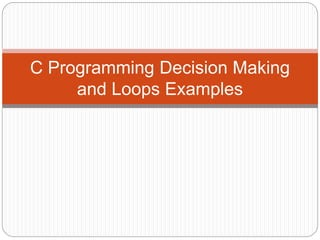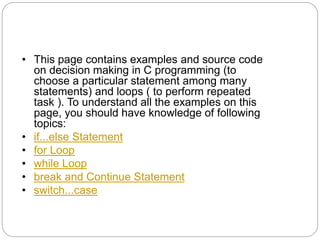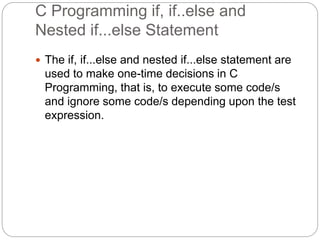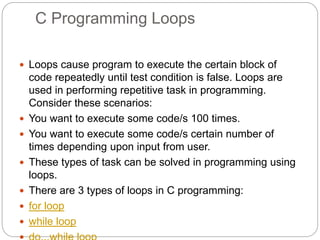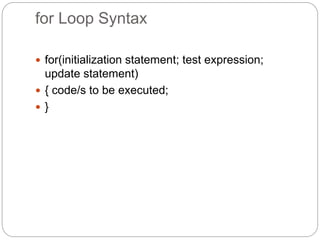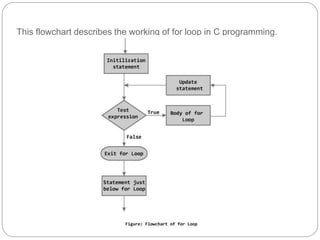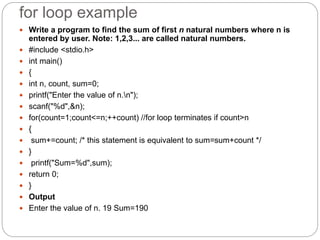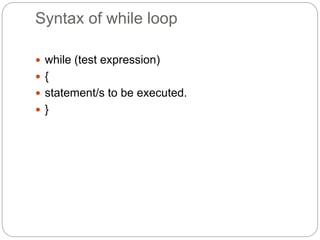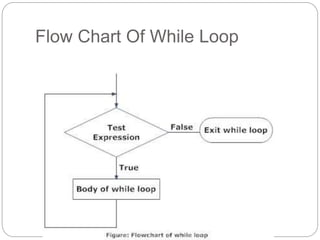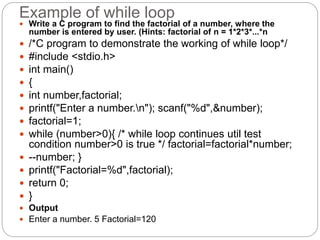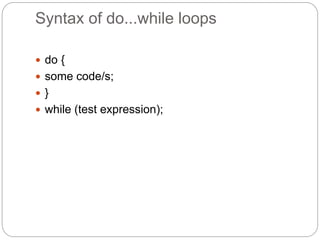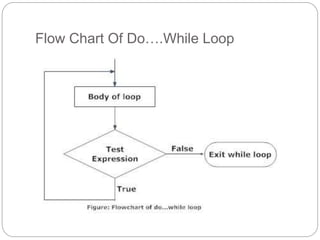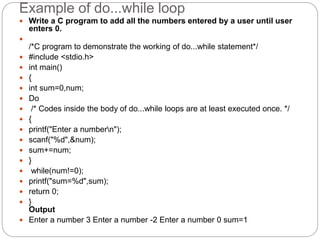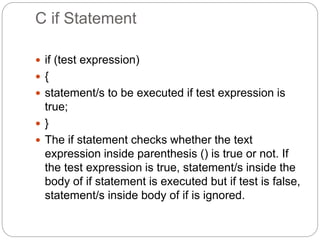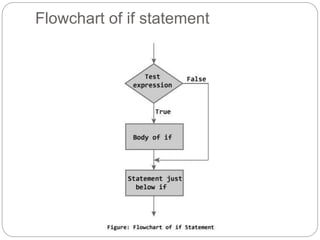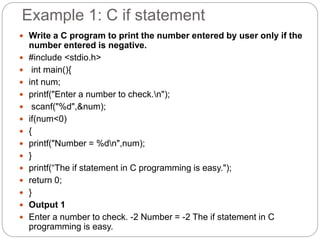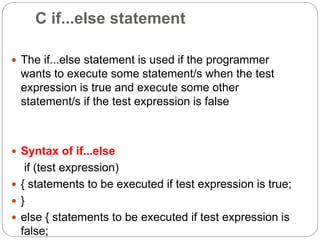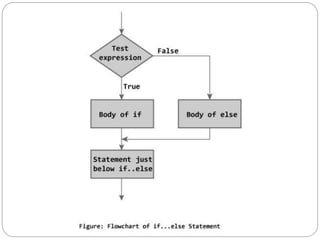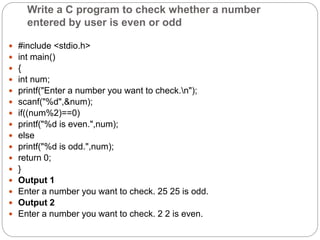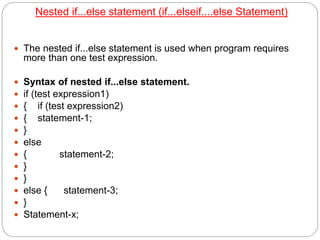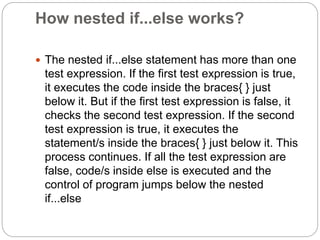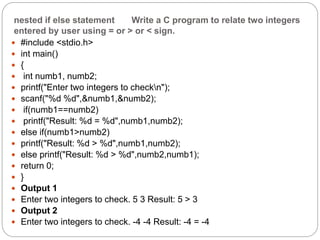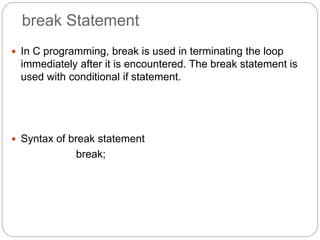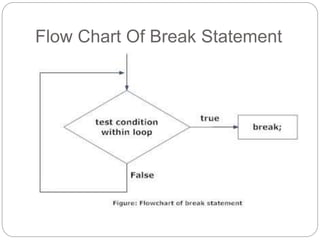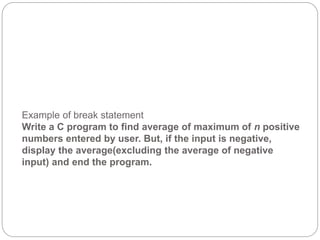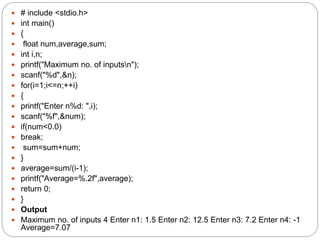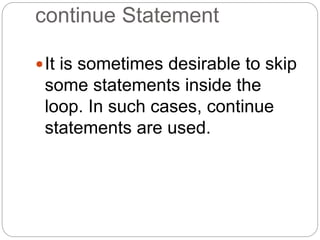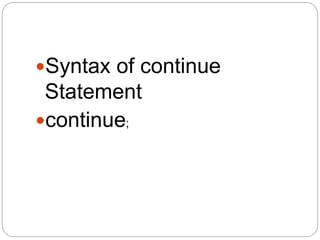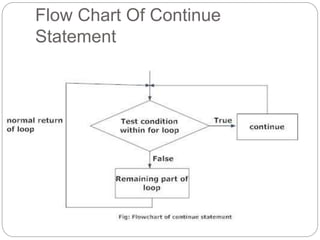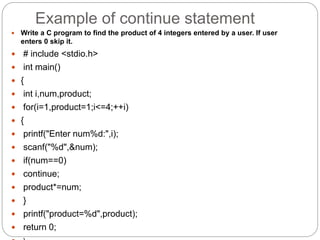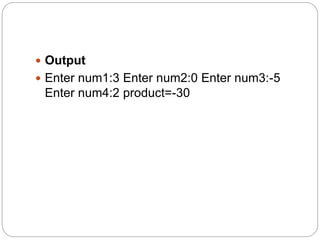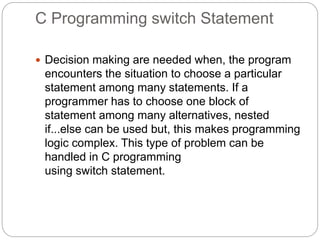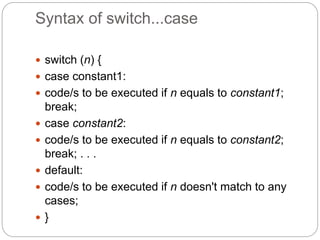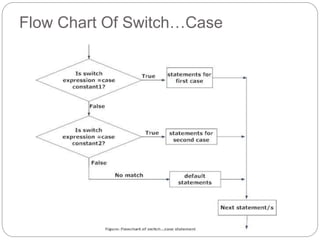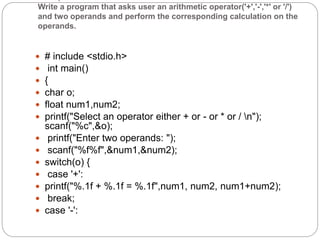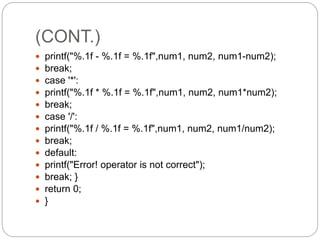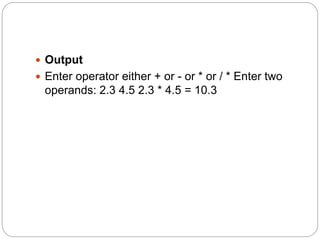1 de 39
Anúncio

### C decision making and looping.

1. welcome
2. C Programming Decision Making and Loops Examples
3. • This page contains examples and source code on decision making in C programming (to choose a particular statement among many statements) and loops ( to perform repeated task ). To understand all the examples on this page, you should have knowledge of following topics: • if...else Statement • for Loop • while Loop • break and Continue Statement • switch...case
4. C Programming if, if..else and Nested if...else Statement  The if, if...else and nested if...else statement are used to make one-time decisions in C Programming, that is, to execute some code/s and ignore some code/s depending upon the test expression.
5. C Programming Loops  Loops cause program to execute the certain block of code repeatedly until test condition is false. Loops are used in performing repetitive task in programming. Consider these scenarios:  You want to execute some code/s 100 times.  You want to execute some code/s certain number of times depending upon input from user.  These types of task can be solved in programming using loops.  There are 3 types of loops in C programming:  for loop  while loop
6. for Loop Syntax  for(initialization statement; test expression; update statement)  { code/s to be executed;  }
7. This flowchart describes the working of for loop in C programming.
8. for loop example  Write a program to find the sum of first n natural numbers where n is entered by user. Note: 1,2,3... are called natural numbers.  #include <stdio.h>  int main()  {  int n, count, sum=0;  printf("Enter the value of n.n");  scanf("%d",&n);  for(count=1;count<=n;++count) //for loop terminates if count>n  {  sum+=count; /* this statement is equivalent to sum=sum+count */  }  printf("Sum=%d",sum);  return 0;  }  Output  Enter the value of n. 19 Sum=190
9. Syntax of while loop  while (test expression)  {  statement/s to be executed.  }
10. Flow Chart Of While Loop
11. Example of while loop  Write a C program to find the factorial of a number, where the number is entered by user. (Hints: factorial of n = 1*2*3*...*n  /*C program to demonstrate the working of while loop*/  #include <stdio.h>  int main()  {  int number,factorial;  printf("Enter a number.n"); scanf("%d",&number);  factorial=1;  while (number>0){ /* while loop continues util test condition number>0 is true */ factorial=factorial*number;  --number; }  printf("Factorial=%d",factorial);  return 0;  }  Output  Enter a number. 5 Factorial=120
12. Syntax of do...while loops  do {  some code/s;  }  while (test expression);
13. Flow Chart Of Do….While Loop
14. Example of do...while loop  Write a C program to add all the numbers entered by a user until user enters 0.  /*C program to demonstrate the working of do...while statement*/  #include <stdio.h>  int main()  {  int sum=0,num;  Do  /* Codes inside the body of do...while loops are at least executed once. */  {  printf("Enter a numbern");  scanf("%d",&num);  sum+=num;  }  while(num!=0);  printf("sum=%d",sum);  return 0;  } Output  Enter a number 3 Enter a number -2 Enter a number 0 sum=1
15. C if Statement  if (test expression)  {  statement/s to be executed if test expression is true;  }  The if statement checks whether the text expression inside parenthesis () is true or not. If the test expression is true, statement/s inside the body of if statement is executed but if test is false, statement/s inside body of if is ignored.
16. Flowchart of if statement
17. Example 1: C if statement  Write a C program to print the number entered by user only if the number entered is negative.  #include <stdio.h>  int main(){  int num;  printf("Enter a number to check.n");  scanf("%d",&num);  if(num<0)  {  printf("Number = %dn",num);  }  printf(“The if statement in C programming is easy.");  return 0;  }  Output 1  Enter a number to check. -2 Number = -2 The if statement in C programming is easy.
18. C if...else statement  The if...else statement is used if the programmer wants to execute some statement/s when the test expression is true and execute some other statement/s if the test expression is false  Syntax of if...else if (test expression)  { statements to be executed if test expression is true;  }  else { statements to be executed if test expression is false;
19. Write a C program to check whether a number entered by user is even or odd  #include <stdio.h>  int main()  {  int num;  printf("Enter a number you want to check.n");  scanf("%d",&num);  if((num%2)==0)  printf("%d is even.",num);  else  printf("%d is odd.",num);  return 0;  }  Output 1  Enter a number you want to check. 25 25 is odd.  Output 2  Enter a number you want to check. 2 2 is even.
20. Nested if...else statement (if...elseif....else Statement)  The nested if...else statement is used when program requires more than one test expression.  Syntax of nested if...else statement.  if (test expression1)  { if (test expression2)  { statement-1;  }  else  { statement-2;  }  }  else { statement-3;  }  Statement-x;
21. How nested if...else works?  The nested if...else statement has more than one test expression. If the first test expression is true, it executes the code inside the braces{ } just below it. But if the first test expression is false, it checks the second test expression. If the second test expression is true, it executes the statement/s inside the braces{ } just below it. This process continues. If all the test expression are false, code/s inside else is executed and the control of program jumps below the nested if...else
22. nested if else statement Write a C program to relate two integers entered by user using = or > or < sign.  #include <stdio.h>  int main()  {  int numb1, numb2;  printf("Enter two integers to checkn");  scanf("%d %d",&numb1,&numb2);  if(numb1==numb2)  printf("Result: %d = %d",numb1,numb2);  else if(numb1>numb2)  printf("Result: %d > %d",numb1,numb2);  else printf("Result: %d > %d",numb2,numb1);  return 0;  }  Output 1  Enter two integers to check. 5 3 Result: 5 > 3  Output 2  Enter two integers to check. -4 -4 Result: -4 = -4
23. break Statement  In C programming, break is used in terminating the loop immediately after it is encountered. The break statement is used with conditional if statement.  Syntax of break statement break;
24. Flow Chart Of Break Statement
25. Example of break statement Write a C program to find average of maximum of n positive numbers entered by user. But, if the input is negative, display the average(excluding the average of negative input) and end the program.
26.  # include <stdio.h>  int main()  {  float num,average,sum;  int i,n;  printf("Maximum no. of inputsn");  scanf("%d",&n);  for(i=1;i<=n;++i)  {  printf("Enter n%d: ",i);  scanf("%f",&num);  if(num<0.0)  break;  sum=sum+num;  }  average=sum/(i-1);  printf("Average=%.2f",average);  return 0;  }  Output  Maximum no. of inputs 4 Enter n1: 1.5 Enter n2: 12.5 Enter n3: 7.2 Enter n4: -1 Average=7.07
27. continue Statement It is sometimes desirable to skip some statements inside the loop. In such cases, continue statements are used.
28. Syntax of continue Statement continue;
29. Flow Chart Of Continue Statement
30. Example of continue statement  Write a C program to find the product of 4 integers entered by a user. If user enters 0 skip it.  # include <stdio.h>  int main()  {  int i,num,product;  for(i=1,product=1;i<=4;++i)  {  printf("Enter num%d:",i);  scanf("%d",&num);  if(num==0)  continue;  product*=num;  }  printf("product=%d",product);  return 0;
31.  Output  Enter num1:3 Enter num2:0 Enter num3:-5 Enter num4:2 product=-30
32. C Programming switch Statement  Decision making are needed when, the program encounters the situation to choose a particular statement among many statements. If a programmer has to choose one block of statement among many alternatives, nested if...else can be used but, this makes programming logic complex. This type of problem can be handled in C programming using switch statement.
33. Syntax of switch...case  switch (n) {  case constant1:  code/s to be executed if n equals to constant1; break;  case constant2:  code/s to be executed if n equals to constant2; break; . . .  default:  code/s to be executed if n doesn't match to any cases;  }
34. Flow Chart Of Switch…Case
35. Write a program that asks user an arithmetic operator('+','-','*' or '/') and two operands and perform the corresponding calculation on the operands.  # include <stdio.h>  int main()  {  char o;  float num1,num2;  printf("Select an operator either + or - or * or / n"); scanf("%c",&o);  printf("Enter two operands: ");  scanf("%f%f",&num1,&num2);  switch(o) {  case '+':  printf("%.1f + %.1f = %.1f",num1, num2, num1+num2);  break;  case '-':
36. (CONT.)  printf("%.1f - %.1f = %.1f",num1, num2, num1-num2);  break;  case '*':  printf("%.1f * %.1f = %.1f",num1, num2, num1*num2);  break;  case '/':  printf("%.1f / %.1f = %.1f",num1, num2, num1/num2);  break;  default:  printf("Error! operator is not correct");  break; }  return 0;  }
37.  Output  Enter operator either + or - or * or / * Enter two operands: 2.3 4.5 2.3 * 4.5 = 10.3
Anúncio